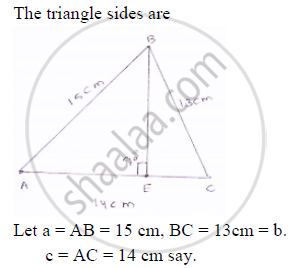# In a δAbc, Ab = 15 Cm, Bc = 13 Cm and Ac = 14 Cm. Find the Area of δAbc and Hence Its Altitude on Ac ? - Mathematics

In a ΔABC, AB = 15 cm, BC = 13 cm and AC = 14 cm. Find the area of ΔABC and hence its altitude on AC ?

#### SolutionNow ,

2s+a+b+c

⇒S=1/2(a+b+c)

⇒s=((15+13+14)/2)cm

⇒=21cm

∴area of a triangle= sqrt(s(s-a)(s-b)(s-c))

=sqrt(21(21-15)(21-14)(21-13)) cm^2

=sqrt(21xx6xx8xx7)cm^2

=84cm^2

Let BE be perpendicular (⊥er) to AC
Now, area of triangle = 84 cm^2

⇒1/2xxBExxACxx84

⇒BE=(84xx2)/(AC)

BE=168/14=12cm

∴Length  of altitude on AC is 12 cm

Concept: Area of a Triangle
Is there an error in this question or solution?

#### APPEARS IN

RD Sharma Mathematics for Class 9
Chapter 17 Heron’s Formula
Exercise 17.1 | Q 4 | Page 8
Share